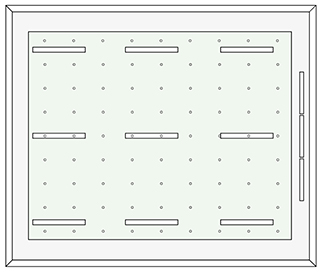# Number of calculation points

EA grid of calculation points should be created to calculate and check the average (mean) illuminance and uniformity of illuminance in the working area, the immediate surroundings and the peripheral surroundings.

The minimum number of calculation points to be used can be calculated using the formula shown below.

Note that the distances and location of the calculation points should not coincide with the distance between the luminaires in the room. A grid that approaches the shape of a box or square is to be preferred, and the relationship between the distance of the length and width of the grid should be kept within the range 0.5–2.

The formula for calculating the maximum distance between calculation points in the grid is as follows:

P = 0,2×5 log d

Where p < 10

d – is the longer length of the surface

p – is the maximum distance between the calculation points

A band of 0.5 meters from each external wall can be excluded from the calculation except when a working area falls within this zone. The size of the grid for walls and ceilings is calculated in a similar way, and here, too, a band of 0.5 m can be excluded from the respective outer edge.

Below is a list of examples of maximum distances between calculation points according to the above formula, based on the size and length of the working area.

Length of the surface
Maximum distance between calculation points
0,40 m 0,15 m or at least 3 points
0,60 m 0,20 m or at least 3 points
1,00 m 0,20 m or at least 5 points
2,00 m 0,30 m or at least 6 points
5,00 m 0,60 m or at least 8 points
10,00 m 1,00 m or at least 10 points
25,00 m 2,00 m or at least 12 points
50,00 m 3,00 m or at least 17 points
100,00 m 5.00 m or at least 20 points

Examples of the number of calculation points for the control of illuminance and uniformity in classrooms and office workplacesWorking area in a standard classroom, without workplaces next to walls.

Supplement with a separate working area within the wall zone. The working area and immediate surroundings should be checked.

Defined working area of 0.6 m in a cellular office with immediate surroundings as a band around the working area with a width of at least 0.5 m. In addition to this, peripheral surroundings as a band of at least 3 m around the immediate surroundings, except that where the peripheral surroundings touch a wall, the area of the peripheral surroundings is restricted by a zone 0.5 m from the walls of the room.